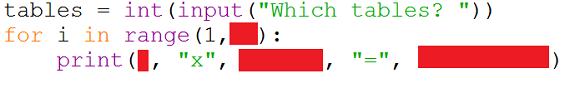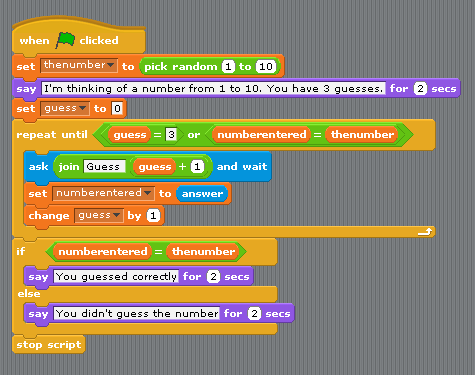# Python For GCSE Section C Exercises

Write a program that prompts the user for a message and a number. Your program should use a for loop to output the message the number of times that the user requests.Write a program that prompts the user for a number from 1 to 12. The full multiplication tables should be displayed for that number.

For example,
User enters 5.
5 x 1 = 5
5 x 2 = 10
etc.

Your task here includes a specific format for the output. You must attempt to output the information as described.

It's a simple program with a small number of things to get right.Here is some pseudocode describing how to calculate the total, largest, smallest and mean average of 10 integers entered by the user.

```asum = 0 amin = 0 amax = 0 n = 0 FOR i = 1 To 10    n = GET THE USER TO ENTER A NUMBER    asum = asum + n    if i ==1:       amin = n       amax = n    if n<amin:       amin = n    if n>amax:       amax = n mean = asum / 10 print asum, mean, amax, amin```

You have to be careful with this. You are printing the mean only once. There is a lot of indentation to get spot on to make this work correctly.

Write a program that asks the user to enter a number. Use loops in your code to work out the sum of the cubes of all of the numbers from 1 to that number. For example, if the user enters 3,

Sum Of Cubes 3: 1 + 8 + 27 = 36

Make a program that will work out if a number is prime or not. The modulus operator tells you the remainder when the first number is divided by the second. The modulus operator in Python is %. ```INPUT n IF n < 2 THEN    OUTPUT "Not Prime" ELSE IF N == 2 THEN    OUTPUT "Prime" ELSE    isPrime = True    FOR i = 2 To n // 2       IF n MODULUS i == 0 THEN isPrime = False    NEXT i    IF isPrime THEN       OUTPUT "Prime"    ELSE       OUTPUT "Not Prime"    END IF END IF```

Write a Python program which iterates the integers from 1 to 50. For multiples of three print "Fizz" instead of the number and for the multiples of five print "Buzz". For numbers which are multiples of both three and five print "FizzBuzz".

Sample Output :

1
2
fizz
4
buzz

```FOR i = 1 TO 50    IF i MODULUS 3==0 AND i MODULUS 5==0 THEN       Print "Fizzbuzz"    ELSE IF i MODULUS 3 == 0 THEN       Print "Fizz"    ELSE IF i MODULUS 5 == 0 THEN       Print "Buzz"    ELSE       Print i    END IF END FOR```

Again, this is pseudocode. Python does not have an END IF or END FOR statement. Python uses indentation to mark the end of these structures. It is normal (and clearer) to write these things when writing pseudocode.

Write a program that produces the following output.

*****
*
*
*****
*
*
*****

You can do this with a single FOR loop counting from 0 to 6 (inclusive). An IF statement inside the loop can be used to determine whether to print 5 asterisks or just 1. There is something special about the numbers of the lines which have 5 asterisks. Work out what links them and you can write this very efficiently.

Write a program to output the following pattern,

1
22
333
4444
55555
666666
7777777
88888888
999999999

Use a nested loops to complete this task.

Write a Python program using loops, to print the following pattern.

*
* *
* * *
* * * *
* * * * *
* * * *
* * *
* *
*

For this program, you will need to use nested loops. A nested loop is a loop that is inside another loop. You will need to draw the first 5 lines of the pattern first, then do the remaining ones.

```FOR i = 1 TO 5    FOR j = 1 TO i       Print "*" (no line break)    END FOR    print line break END FOR FOR i = 4 TO 1    FOR j = 1 TO i       Print "*" (no line break)    END FOR    print line break END FOR```

The above is pseudocode. Take the trouble to check how to write the FOR loops correctly. Take care when counting backwards – you have an example to use to guide you.

Write a Python program that accepts an integer (n) and computes the value of n+nn+nnn.

Sample value of n is 5
5 + 55 + 555 = 615
Expected Result: 615

```Hint n =5 nn =n*10+n```

This is a very old algorithm first described by Euclid in around 300BC. It calculate the highest common factor of two numbers.

```input a input b while a not equal to b    If a>b Then       Set a to a-b    End if    If b>a Then       Set b to b-a    End if end while Set hcf variable to a Output hcf```

Rewrite the following Scratch program in Python. The Repeat...Until needs to be replaced with a while loop. When you change UNTIL to WHILE, you are inverting the conditions.To determine if a number is a palindrome, you need a way to reverse it. There are some cheaty, cheaty ways to do this easily.

A much cooler way is to use arithmetic.

For example,

• Take the number, 123.
• To get the rightmost digit, find the modulus (remainder) when that number is divided by 10 (n % 10).
• If we floor divide by 10 (divide and round down – n // 10), we can remove the last digit. This gives us 12.
• When we take the 2, we want to add it to the right end of the number we started with 3. Multiply by 10 and add our 2. That gives 32.
• We repeat this process until our original number is 0.
```n = integer INPUT "Enter your number: " tmp = n rev = 0 WHILE tmp>0    rev = (10 x rev) + tmp MOD 10    tmp = tmp DIV 10 END WHILE IF n == rev THEN    OUTPUT n, "is a palindrome." ELSE    OUTPUT n, "is not a palindrome." END IF```

A palindromic number reads the same both ways. The largest palindrome made from the product of two 2-digit numbers is 9009 = 91 × 99.

Find the largest palindrome made from the product of two 3-digit numbers. You can adapt your previous program to do this.

```largest = 0 FOR a = 999 to 100 STEP -1    FOR b = 999 to 100 STEP -1       n = a x b       tmp = n       WHILE tmp > 0          rev = (10 x rev) + tmp MOD 10          tmp = tmp DIV 10       END WHILE       IF rev = n and n>largest THEN          largest = n       END IF    END FOR END FOR OUTPUT largest```

Working from left to right, a number is an increasing number if each digit is not exceeded by any of the digits to the left. For example,

134468

• Use only positive integers.
• Assume that single digit numbers are increasing numbers.

1. What proportion of the numbers up to and including 1000 are increasing numbers?
2. What proportion of the numbers up to and including 10000 are increasing numbers?

Repeat the previous task but for decreasing numbers. Assume that all single digit numbers are decreasing numbers.

Here is an alternative for the Euclidean highest common factor algorithm.

```Set a variable to what the user enters Set b variable to what the user enters WHILE b not equal to 0    t = b    b = a MOD b    a = t END WHILE Output a```

Write program to calculate the lowest common multiple of 2 numbers entered by the user. You will need to combine HCF code with this program.

The while loop changes the value of a and b. Make 2 variables x and y and make them equal to a and b before the while loop.

x = a
y = b

Then use the following formula to calculate the LCM.

lcm = (x * y) / hcf

Use the code from one of your HCF programs and add a line to calculate the LCM of the two numbers and another to output this LCM.